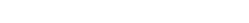# BizTalk maps with two inputs – Complement, Intersect and Union of Sets

Posted: November 24, 2012  |  Categories: BizTalk Uncategorized

Union

In set theory, the union (denoted by ∪) of a collection of sets is the set of all distinct elements in the collection. It is one of the fundamental operations through which sets can be combined and related to each other. (http://en.wikipedia.org/wiki/Union_(set_theory)).For example, if A = {1, 2, 3, 4, 5, 6, 7} and B = {2, 3, 4} then AB = {1, 2, 3, 4, 5, 6, 7}.  A BizTalk map example would contain two input messages and merge them in to one output that contains all values. If  the two input messages are as show below

<ns0:Root xmlns:ns0=”http://schemas.microsoft.com/BizTalk/2003/aggschema”>
<InputMessagePart_0>
<ns1:Tags xmlns:ns1=”http://Complement_Map_Test.Tags”>
<Replacement>Replacement_0</Replacement>
<Tag>
<TagNumber>2</TagNumber>
</Tag>
<Tag>
<TagNumber>3</TagNumber>
</Tag>
<Tag>
<TagNumber>4</TagNumber>
</Tag>
</ns1:Tags>
</InputMessagePart_0>
<InputMessagePart_1>
<ns1:Tags xmlns:ns1=”http://Complement_Map_Test.Tags”>
<Replacement>Replacement_0</Replacement>
<Tag>
<TagNumber>1</TagNumber>
</Tag>
<Tag>
<TagNumber>2</TagNumber>
</Tag>
<Tag>
<TagNumber>3</TagNumber>
</Tag>
<Tag>
<TagNumber>4</TagNumber>
</Tag>
<Tag>
<TagNumber>5</TagNumber>
</Tag>
<Tag>
<TagNumber>6</TagNumber>
</Tag>
<Tag>
<TagNumber>7</TagNumber>
</Tag>
</ns1:Tags>
</InputMessagePart_1>
</ns0:Root>

The output of a union is

<ns0:Tags xmlns:ns0=”http://Complement_Map_Test.Tags”>
<Tag>
<TagNumber>1</TagNumber>
</Tag>
<Tag>
<TagNumber>2</TagNumber>
</Tag>
<Tag>
<TagNumber>3</TagNumber>
</Tag>
<Tag>
<TagNumber>4</TagNumber>
</Tag>
<Tag>
<TagNumber>5</TagNumber>
</Tag>
<Tag>
<TagNumber>6</TagNumber>
</Tag>
<Tag>
<TagNumber>7</TagNumber>
</Tag>
</ns0:Tags>

An example of a map that does this union without creating duplicates is shown belowThis logic builds upon logic in another blog called SELECT DISTINCT in a BizTalk Map. The first scripting functoid ,creates a global list variable and is attached to the root node. Since the script has no output and root node is always output, we don’t need to block anything.

public System.Collections.Generic.List<int> excludedList = new System.Collections.Generic.List<int>();

public void DeclareExludedList()
{}

The second and third scripting functoid compares the list content with the current node content on each loop. It returns true if the current content can be found in the list or it adds the content to the list and then returns false.  True is an intersecting content whereas false is complementing content. The output is tested by the Logical Equal functoid following it,  and if it is false it will become an output record. This is achieved by connecting the Logical Equal output directly to the destination NODE, effectively filtering the input node-list.

public bool IsInteresect( int TagNumber )
{
if( excludedList.Contains( TagNumber ) )
return true;
return false;
}

Complement

In set theory, a complement of a set A refers to things not in (that is, things outside of) A. The relative complement of A with respect to a set B, is the set of elements in B but not in A. When all sets under consideration are considered to be subsets of a given set U, the absolute complement of A is the set of all elements in U but not in A.(http://en.wikipedia.org/wiki/Complement_(set_theory))For example, if A = {1,2,3,4, 5, 6, 7} and B = { 2, 3, 4} then A \ B = {1, 5, 6, 7}. A BizTalk map example would contain two input messages and merge them into one output that only contains the relative complement. If we used the same two input messages as in the union example above then the output would be;

<ns0:Tags xmlns:ns0=”http://Complement_Map_Test.Tags”>
<Tag>
<TagNumber>1</TagNumber>
</Tag>
<Tag>
<TagNumber>5</TagNumber>
</Tag>
<Tag>
<TagNumber>6</TagNumber>
</Tag>
<Tag>
<TagNumber>7</TagNumber>
</Tag>
</ns0:Tags>

An example of a map that does this relative complement  is shown belowThe first scripting functoid creates the same global list variable as in the union example.

The second scripting functoid adds all the content from the first input to global list variable and has no output. A logical string is used to block output to node on the output schema. A node in the output that occurs before node the contains the complement is used to make sure that the list is populated before the complement transform is  run.

public void add2ExcludedList( int TagNumber )
{
}

The third scripting functoid and logical equality functoid are the same  as that used in the third functoid in the union example above.

Intersect

The intersection (denoted as ∩) of two sets A and B is the set that contains all elements of A that also belong to B (or equivalently, all elements of B that also belong to A), but no other elements.(http://en.wikipedia.org/wiki/Intersection_(set_theory)).For example, if A = {1, 2, 3, 4, 5, 6, 7} and B = {2, 3, 4} then AB = {2, 3, 4}. A BizTalk map example would contain two input messages and merge them in to one output that contains the complement. If we used the same two input messages as in the union example above then the output would be;

<ns0:Tags xmlns:ns0=”http://Complement_Map_Test.Tags”>
<Tag>
<TagNumber>2</TagNumber>
</Tag>
<Tag>
<TagNumber>3</TagNumber>
</Tag>
<Tag>
<TagNumber>4</TagNumber>
</Tag>
</ns0:Tags>

A map that does this intersect is the same as the complement example above except the that logical equality tests for true rather than false.

The examples above are examples of how you might use two inputs in map and utilise global variables that are lists. Sample code that was used here can be found at Complement Map Test.zipOver 500+ customers across
30+ countries depend on BizTalk360Manage and monitor serverless
components effortlessly